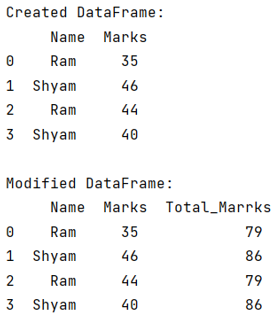# How to create a new column from the output of pandas groupby().sum()?

Given Pandas DataFrame, we have to create a new column from the output of the given Pandas groupby().sum().
Submitted by Pranit Sharma, on July 01, 2022

Pandas is a special tool that allows us to perform complex manipulations of data effectively and efficiently. Inside pandas, we mainly deal with a dataset in the form of DataFrame. DataFrames are 2-dimensional data structures in pandas. DataFrames consist of rows, columns, and data.

DataFrame can be created with the help of Python dictionaries or lists, but in the real world, CSV files are imported and then converted into DataFrames.

## Create a new column from the output of pandas groupby().sum()

To create a new column for the output of groupby.sum(), we will first apply the groupby.sim() operation and then we will store this result in a new column.

### groupby().sum()

On the other hand, groupby() is a simple but very useful concept in pandas. By using groupby(), we can create a grouping of certain values and perform some operations on those values.

Groupby function split the object, apply some operations, and then combines them to create a group hence large amount of data and computations can be performed on these groups. One of the simplest methods on groupby objects is the sum() method.

To work with pandas, we need to import pandas package first, below is the syntax:

```import pandas as pd
```

Let us understand with the help of an example,

## Python program to create a new column from the output of pandas groupby().sum()

```# Importing pandas package
import pandas as pd

# Creating a DataFrame
df = pd.DataFrame({
'Name':['Ram','Shyam','Ram','Shyam'],
'Marks':[35,46,44,40]
})

# Display DataFrame
print("Created DataFrame:\n",df,"\n")

# Applying groupby and sum and storing it into a new column
df['Total_Marrks'] = df.groupby('Name', sort=False)['Marks'].transform('sum')

# Display modified DataFrame
print("Modified DataFrame:\n",df)
```

## Output